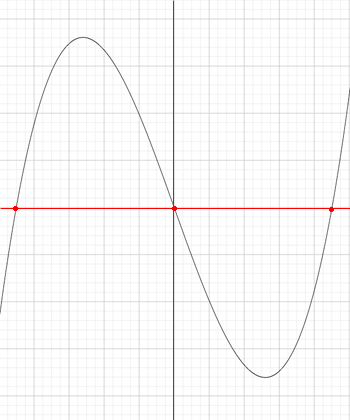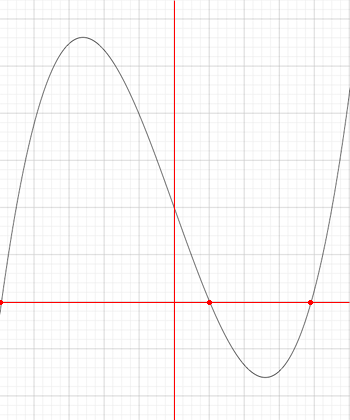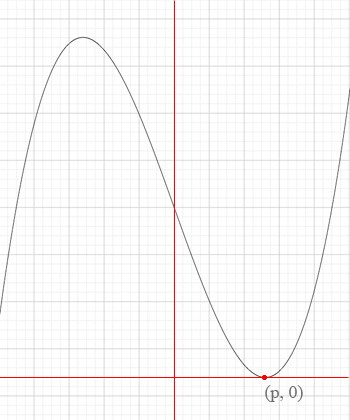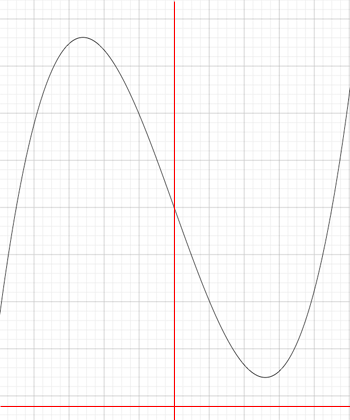SEARCH HOMEMath Central Quandaries & QueriesQuestion from Jyotishman, a student: What can be the value of a for the equation to have 3 roots? x^3-13x+a=0Hi Jyotishman,

This problem involves geometry and calculus. The geometry tells me what it is I need to find and the calculus gives me a method to find it. I can get you started.

First I graphed y = f(x) = x3 - 13x + a with a = 0. The axes are in red and I didn't put the scale on the axes since I am interested in the shape not the scale. This graph crosses the x-axis in 3 places marked by the red dots.As a increases he graph moves upwards relative to the axes maintaining three distinct zeros untiluntil a attains a value for which the polynomial f(x) = x3 - 13x + a two distinct roots, one of them I am going to call p as in the diagram. (One root is not on my diagram.) OIf a increases beyond this value the resulting graph only crosses the x-axis at one point (not on the diagram).The task is thus to find p and then the value of a so that f(p) = p3 - 13p + a = 0.

p is a critical value for the polynomial f(x) so it can be found by solving f '(x) = 0.

Do you now see how to complete the problem? If you need further assistance write back.

HarleyMath Central is supported by the University of Regina and The Pacific Institute for the Mathematical Sciences.